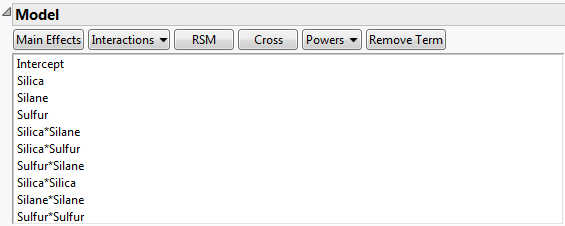Publication date: 08/13/2020

## Model

What appears in the Model outline depends upon whether the associated data table contains a Model or Fit Model script:

If there is a script, the Model outline contains the estimable effects specified in the script. Effects are entered into the model in the order specified in the script. If the model script contains more effects than are estimable, those that are not estimable are not included in the model for evaluation.

If there is no script, the Model outline contains only main effects.

Figure 15.13 shows the Model outline for the Bounce Data.jmp data table, found in the Design Experiment folder. The Model script in the data table contains response surface effects for the three factors Silica, Silane, and Sulfur. Consequently, the Model outline contains the main effects, two-way interactions, and quadratic effects for these three factors.

Figure 15.13 Model Outline for Bounce Data.jmpYou can add effects to the Model outline using the following buttons:

Main Effects

Adds main effects for all factors in the model.

Interactions

Adds interaction effects. If no factors are selected in the Factors outline, select 2nd, 3rd, 4th, or 5th to add all appropriate interactions up to that order. Add interactions up to a given order for specific factors by selecting the factor names in the Factors outline, selecting Interactions, and then specifying the appropriate order. Interactions between non-mixture and mixture factors, and interactions with blocking and constant factors, are not added.

RSM

Adds interaction and quadratic terms up to the second order (response surface model terms) for continuous factors. Categorical factors are not included in RSM terms. Main effects for non-mixture factors that interact with all the mixture factors are removed.

Cross

Adds specific interaction terms. Select factor names in the Factors outline and effect names in the Model outline. Click Cross to add the crossed terms to the Model outline.

Powers

Adds polynomial terms. If no factor names are selected in the Factors outline, adds polynomial terms for all continuous factors. If factor names are selected in the Factors outline, adds polynomial terms for only those factors. Select 2nd, 3rd, 4th, or 5th to add polynomial terms of that order.

Scheffé Cubic

Adds Scheffé cubic terms for all mixture factors. These terms are used to specify a mixture model with third-degree polynomial terms.

Remove Term

Removes selected effects.